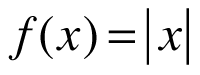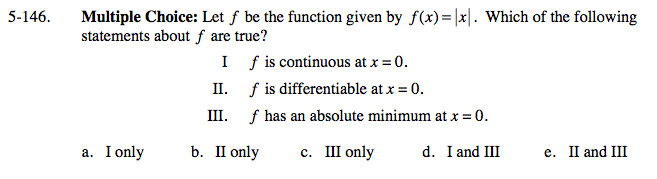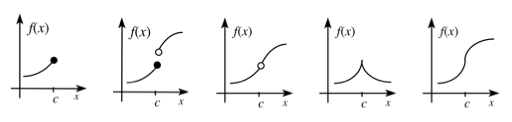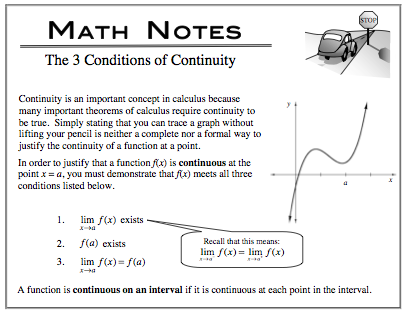### Home > CALC > Chapter 5 > Lesson 5.4.1 > Problem5-146

5-146.
1. Choice: Let f be the function given by. Which of the following statements about f are true? Homework Help ✎

2. I. f is continuous at x = 0.
II. f is differentiable at x = 0.
III. f has an absolute minimum at x = 0.

1. I only

2. II only

3. III only

4. I and III

5. II and IIIExamples of functions that are not differentiable at x = c. Notice that some of them are still continuous at x = c.